# The Finite Element Method¶

Practical session during the Daedalus Project P8: Regression for uncertainty quantification in PDE's with stochastic coefficients, November, 2018.

## Stationary Diffusion¶

Given a Lipschitz domain $D\subseteq\mathbb{R}^n$ the stationary diffusion equation with homogeneous Dirichlet boundary conditions, diffusion coefficient $\kappa$ and forcing term $f$ reads: \begin{equation} \label{eq:pde} \begin{aligned} -\operatorname{div} (\kappa \nabla u) &= f &\text{in } D, \\ u &= 0 &\text{on } \partial D \end{aligned} \tag{1} \end{equation} This equation is the prime example for a linear elliptic partial differential equation (PDE). In this notebook we will employ the finite element method to threat this problem numerically. For this we will use Python and the FEniCS library.

## Solution¶

Not every PDE admits a solution in the classical sense. A function $u$ is called

• a classical solution of $(1)$ if it satisfies the equation exactly,
• a strong solution of $(1)$ if \begin{align} \int_D - \operatorname{div}(\kappa \nabla u) v\ \mathrm{d}\lambda &= \int_D f v\ \mathrm{d}\lambda & \text{for all } v\in C^0(D) \end{align}
• and a weak solution of equation $(1)$ if \begin{align} \int_D \kappa \nabla u \cdot\nabla v\ \mathrm{d}\lambda &= \int_D f v\ \mathrm{d}\lambda & \text{for all } v\in C_0^1(D) \end{align} with $C_0^k(D) := \left\{ w\in C^k(D) | w = 0 \text{ on } \partial D \right\}.$

Remark: Each solution concept has its own requirements on the regularity of $\kappa$, $f$ and $u$. But note that a strong solution $u$ satisfies the equation almost everywhere and that the concept of a weak solution can be derived from that of a strong solution by the integration by parts formula.

Recall that the Sobolev space $H^1(D)$ is defined as the space of functions in $L^2(D)$ such that their distributional derivative has a representation in $L^2(D)$ as well. $H^1(D)$ is a Hilbert space with inner product $$\langle f, g\rangle_{H^1(D)} = \int_D \left( fg + \nabla f \cdot \nabla g \right) \mathrm{d}x.$$ A weak solution can then be sought for in the closed subspace $H^1_0(D) \subseteq H^1(D)$ which is defined to be the closure of $C^\infty_0(D)$ with respect to the $H^1(D)$-norm.

To formalise this we define the bilinear form $a : H^1_0(D) \times H^1_0(D) \to \mathbb{R}$ and the linear form $L : H^1_0(D) \to \mathbb{R}$ as $$a(u,v) := \int_D \langle \kappa \nabla u, \nabla v\rangle\ \mathrm{d}\lambda \qquad\text{and}\qquad L(v) := \int_D f v\ \mathrm{d}\lambda$$ and rewrite the equation as $$a(u,v) = L(v) \qquad\text{for all } v \in V := H^1_0(D). \tag{2}$$ Note that the function space $H^1_0(D)$ is chosen in such a way that $u = 0$ on $\partial D$ is ensured by definition in the sense of traces.

If $\kappa\in L^{\infty}(D)$ and $\kappa \ge \varepsilon > 0$ almost everywhere and $f\in H^{-1}(D)=H_0^1(D)^*$, the Lax-Milgram Theorem ensures the existence and uniqueness of a solution $u$ such that $$\|u\|_{H^1_0(D)} \lesssim C(\varepsilon)\|f\|_{H^{-1}(D)}$$

## Galerkin Projection¶

The finite element method (FEM) tries to approximate the solution of $(2)$ in a suitable finite dimensional function space $V_h \subseteq V$. Since the resulting equation is a linear equation on a finite dimensional vector space it induces a matrix equation for the coefficients of a chosen basis of $V_h$.

## Implementation¶

In :
from fenics import *
% matplotlib inline

In :
import matplotlib as mpl
mpl.rcParams['image.cmap'] = 'viridis'
mpl.rcParams['figure.figsize'] = [3, 3]
mpl.rcParams['figure.dpi'] = 100
mpl.rcParams['lines.linewidth'] = 0.5
mpl.rcParams['text.usetex'] = False
mpl.rcParams['font.size'] = 12


#### FEM Spaces¶

The FE method uses piecewise pull-back functions of polynomials on a given partition of $D$ as the discrete solution spaces. In the case of a (vertex) triangular mesh, a pull-back function is a polynomial on each triangle.

In this example we will use Lagrange Polynomials of degree 1 on an automatically generated mesh. FEniCS allows us to specify the discrete function spaces, (bi)linear forms and boundary conditions in an intuitive way and takes care of the assembly the necessary matrix and vector.

In :
# Create mesh and define function space
mesh = UnitSquareMesh(32, 32)
fe_type = "Lagrange"
fe_degree = 1
V = FunctionSpace(mesh, fe_type, fe_degree)

plot(mesh)

Out:
[<matplotlib.lines.Line2D at 0x7f3c05de2610>,
<matplotlib.lines.Line2D at 0x7f3c0833bfd0>]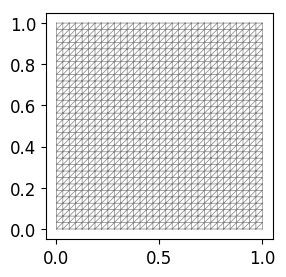In :
# Define boundary condition
u0 = Constant(0.0)
bc = DirichletBC(V, u0, 'on_boundary')

# Define variational problem
u = TrialFunction(V)
v = TestFunction(V)
kappa = Constant(1)
f = Constant(1) # Expression("1", degree=3)
L = f*v*dx

# Compute solution
u = Function(V)
solve(a == L, u, bc)

plot(u)

Out:
<matplotlib.tri.tricontour.TriContourSet at 0x7f3c0524d8d0>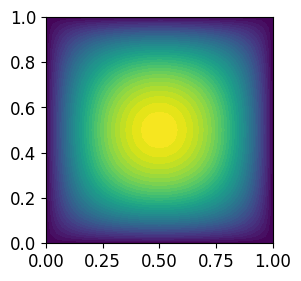Now that we know how to solve the equation we can write a function that returns the solution given the diffusion coefficient $\kappa$ and forcing term $f$ as parameters.

In :
def solution(kappa, f):
u = TrialFunction(V)
v = TestFunction(V)
L = f*v*dx

u = Function(V)
solve(a == L, u, bc)
return u


The diffusion coefficient $\kappa$ can be given as a FEnics function living on some discrete space or as an parametric expression.

In :
kappa = Expression("1 + exp(a * sin(2*pi*x) * cos(2*pi*x))", a=10, degree=3)


Moreover the interpolate routine of FEnics gives function representation on the given space based on interpolation.

In :
kappa_interp = interpolate(kappa,V)
plot(kappa_interp)

Out:
<matplotlib.tri.tricontour.TriContourSet at 0x7f3c04e41690>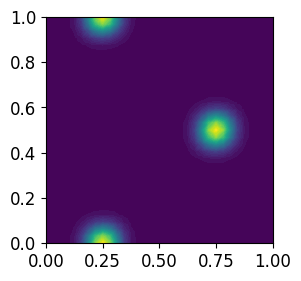Now both versions of $\kappa$ (quadrature/interpolation based) can be used to solve the discrete problem.

In :
u = solution(kappa, f)
plot(u)

Out:
<matplotlib.tri.tricontour.TriContourSet at 0x7f3c048e4990>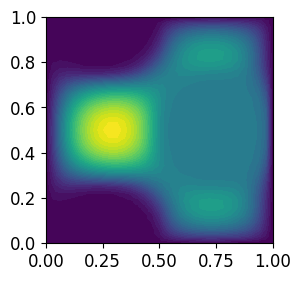In :
u = solution(kappa_interp, f)
plot(u)

Out:
<matplotlib.tri.tricontour.TriContourSet at 0x7f3c0459d490>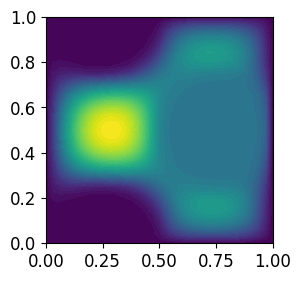End of part I. Next: random fields

© Robert Gruhlke, Manuel Marschall, Phillip Trunschke, 2018-2019